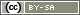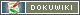# Scale Index

Psychological constructs (e.g. optimism) are sometimes not directly observable. Therefore a scale is often used for measurement in questionnaires: Several separate questions – known as scale items – that all represent different aspects of the same construct.

The more scale items are used to measure the construct, the more accurate is the measurement, because measurement errors for individual items will be less important. The assumption is certainly that the scale was neatly constructed and validated (the construction of scales is a complex process: to provide 100 times the same question, of course, does not provide a better measure than to ask this question only once).

In the analysis the individual answers are reckoned up in order to obtain the mean index or sum index, to quantify the construct. The mean index has two advantages:

• The range of values of a mean value index is the same as for the individual items. If they range from 1 to 5, the mean index lies also between 1 and 5. This makes the interpretation easier.
• A mean index can also be quite easily calculated when individual responses are missing (missing values). However, the mean values of individual items may not vary too much – otherwise missing values lead to measurement artifacts.

## Computation in SPSS

The simplest case is to calculate a mean value index in SPSS using the syntax (File → New → syntax). For sum indices just replace the MEAN by SUM:

```COMPUTE AB01 = MEAN(AB01_01 TO AB01_10).
EXECUTE.```

In the above example the variables from AB01_01 to AB01_10 are calculated to an index. If you want to offset individual items from a scale to a subscale index just separate them with a comma:

```COMPUTE AB01 = MEAN(AB01_01, AB01_03, AB01_05, AB01_07, AB01_09).
EXECUTE.```

These examples would also calculate an average if only one of the items was answered. You can determine with a point behind the MEAN ()-command, that a minimum number of responses per case have to be present. Three quarter of the items is a reasonable minimum value – e.g. 8 of 10 items:

```COMPUTE AB01 = MEAN.8(AB01_01 TO AB01_10).
EXECUTE.```

Reversed polarity items – in other words items which at are negatively worded and therefore agreement indicates a lower manifestation of the construct – should be marked when compiling the questionnaire: Select item in the List of Questions → “Invert answer codes for this item”.

Important: Items with reversed polarity must be marked accordingly before the start of the survey. Do not change this setting during or after the survey because already collected responses will not be recoded.

If you have not inverted your items initially you have to recode them afterwards. In SPSS there are two ways of doing so. The following examples are based on a 5-point scale:

```RECODE AB01_01 (1=5) (2=4) (3=3) (4=2) (5=1) (ELSE=SYSMIS) INTO AB01_01R.
EXECUTE.```
```COMPUTE AB01_01R = 6 - AB01_01.
EXECUTE.```

Caution: In the second more sophisticated version you have to be careful with missing values. If you have a -1 is will be recoded to 7. This version is especially appropriate if the respondents have to answer all questions and if there is no “do not know” option offered.

For the scale index of course the recoded variable must be used:

```COMPUTE AB01 = MEAN(AB01_01R, AB01_02, AB01_03R, AB01_04, AB01_05R, AB01_06, AB01_07R, AB01_08, AB01_09R, AB01_10).
EXECUTE.```

Can I always combine variables to an index?

The calculation of a scale index is only sensible if all items reflect the same construct. In practice you can test this by computing the correlation between the items by means of Cronbach's alpha.

As a rule of thumb Cronbach's alpha should be above .7. However, Cronbach's alpha is highly influenced by the amount of items. Therefore a scale with only 4 or 5 items can be plausible with an alpha value of .6.

Do I have to z-standardize the items before calculating the scale index?

It depends on the scale. The z-standardization has one drawback: The range of the scale index is not the same as for the individual items. This complicates the interpretation: While you know that a score of 2.7 measured with a 5-point scale (1 to 5) is near the center of the scale, the meaning of a z-value of -0.2 is not so readily apparent.

A z-standardization is especially beneficial if you there are missing values in the data. Imagine for example that almost all participants answer one item with “strongly agree”, while the item mean for the other items is near the center of the scale. So if only one participant does not answer the item most probably the score 5 is “missing” – and therefore the scale index is most likely lower than if the participant had answered the item. If the items are z-standardized (or mean-normalized), this source of error is eliminated.

However, the z-standardization normalizes the standard deviation of the individual items. If most of the participants answer one item in the same way (with the same point on the scale), a deviation of one scale-point has more impact after z-standardization as in cases where there is a great variation in the responses of the participants. Therefore some items have a stronger impact on the scale index than others. Whether this is favorable or not depends on the scale. Anyway, in a perfect scale all items would have almost the same mean (centered in the middle of the scale) and the same standard deviation…

Does the correlation of a construct with other constructs depend on the number of items?

Yes and no. In principle the size of the correlation is independent from the number of items. However, in a neatly constructed scale the quality of the measurement is increased by the number of items. Therefore the scale index contains fewer measurement errors and as a result higher correlations may be observed.

On the contrary a correlation based on more items can actually be overrated if both constructs underlie the same measurement error. The higher correlation with more items is a spurious correlation in this case – e.g. because some people prefer to answer on the right end of the scale (acquiescence).

What is the measurement level of scale indices?

Interval scales (metric). For the computation of a mean or a sum you have to assume that you items are at least approximately interval scaled (quasi-metric). Consequently, the scale index is interval scaled.

Are scales with reversed-polarity items preferable?

There is no general answer to this question.

The use of reversed-polarity items will usually result in the effect that the correlation between items (Cronbach's alpha) is slightly lower. Basically that is not desirable – but might as well be an indicator that the respondents have made their answer thoughtfully. In addition, a general tendency to approval/rejection is extenuated by the use of reversed-polarity items. This results in a superior measurement of the construct.

On the other hand, people may answer reversed-polarity items in different ways. For example, someone could avoid to answer “never” because he likes to appear especially honest. This can lead to measurement artifacts. Moreover there is some evidence that reversed-polarity items can have an impact on the unidimensionality of scales.

Mean values or factor values for the scale index?

Especially when a scale battery maps several sub-dimensions/sub-constructs, this question is at hand: For the indices of the subconstructs, should we simply average the items assigned to a subconstruct or instead work with the factor scores from an exploratory factor analysis?

The factor scores incorporate the individual items with different weights. Theoretically, the factor values map the vectors of the subconstructs a bit more accurately in this way. Practically, this advantage is negligible. Practically, factor values are accompanied by a significant disadvantage: The calculation of the indices is a little different in each data set that uses the scale – just depending on exactly how the factors lie. This means that comparability between studies is lost.

Moreover, it should be kept in mind that the concrete factor solution (and thus the weighting) is only one of many possible solutions – and it is in large part also the result of measurement artifacts, the choice of optimization procedure, etc…

The lack of comparability and the influence of measurement errors argue for “normal” mean values. Such an index is usually also theoretically better supported, because in the ideal case it is already a priori clarified which items belong to which subconstruct.